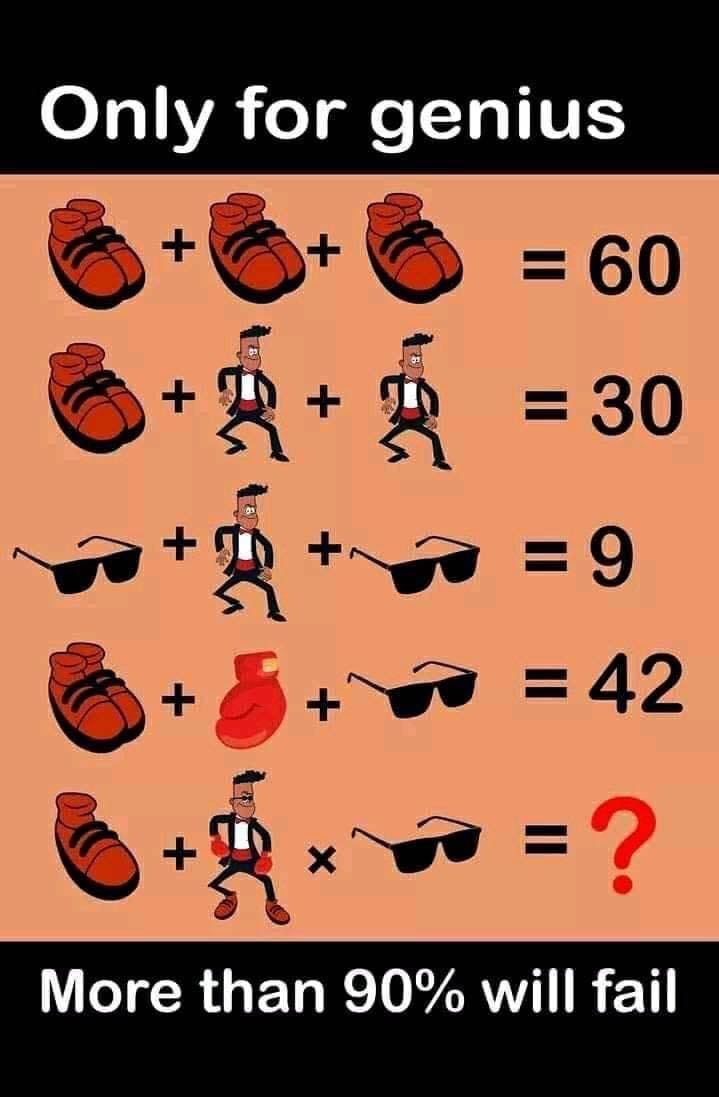This post may contain affiliate links. This means if you click on the link and purchase the item, We will receive an affiliate commission at no extra cost to you. See Our Affiliate Policy for more info.

# The Shoe, Goggles, Man and Boxing gloves Puzzle – Genius Puzzle With Correct Answer

41
79917

### Check out this new genius puzzle on the internet getting viral for a reason. The Shoe, Goggles, Man and Boxing gloves Puzzle. Only for genius puzzle, more than 90% will fail to solve this genius math puzzle.

Can you solve this viral logical math puzzle? Check out the image and share your equation or answer in the comment section below. Best of luck!

### Puzzle Question:

⇒ Shoes + Shoes + Shoes = 60

⇒ Shoes + Man + Man = 30

⇒ Goggles + Man + Goggles = 9

⇒ Shoes + Boxing gloves + Goggles = 42

⇒ Shoes + Man × Goggles = ??

(check the image for details)### Join the Discussion Here:

Check out the solution in this video and don’t forget to share this awesome puzzle in your group.

Source & References

Search items:

The Shoe Goggles Man and Boxing gloves Puzzle, The Shoe Puzzle, Shoes + Shoes + Shoes = 60 Viral Math Puzzle, Hard Math Puzzles Images, Viral Facebook Math Puzzle Image, Viral Whatsapp Image Puzzle, Puzzles for Adults Logical Puzzle Question, Only for Genius Puzzles, Shoes Sunglass Puzzle with answer. Difficult Math puzzle images, Only genius can solve this puzzle,

1.Wissam

48

2.Aadi

154

3.Hajbi

144

4.Lanre

The answer is really 154. Don’t forget to add the “5” of the man putting on the gloves, goggles and the boot.

5.Rajesh

How can you pls explain

6. Kante can you please explain

7.Mara

30

8.Anand

The equation is 10+(20+40+5+2)*2
10+67*2
10+134
=144

9.Wasim

⇒ Shoes20 + Shoes 20+ Shoes20 = 60

⇒ Shoes 20+ Man 5+ Man 5= 30

⇒ Goggles 2+ Man 5+ Goggles 2= 9

⇒ Shoes 20+ Boxing gloves20 + Goggles2 = 42

⇒ Shoes 20+ Shoes 20+Goggles2+Boxing glove20+Man 5× Goggles2 = 134

10.Rahul Bhimte

144

11.Rajitha

20

12.Moksha

30

13.Moksha

154

14.JohnnyRich

30

15.hw

the answer is 20 one shoes 10 a.man 5 and the goggles 2 the equation is 10+(5*2)=20

16.Hina Bhat

I think 140

17.Venkatesh Sherla

18.Venkatesh Sherla

Shoe20+man5+shoes 40+eye2+gloves 40=107
107*2=214

19.tracy

154 (10 + [20+5+2+20+20] ) * 2

20.Nuzhat Jahan

21.Sjkalani

114

22.RAJU

144

23.Teresa

174

24.Juan Clay GodsServant

10+5+2+40+(20×2)=97

25.Harold

27

26.Julia

You guys are all part of the 90% fail.
You’re forgetting the “order of operations” rule. Don’t put brackets in where they don’t belong! You have to multiply the man wearing all the gear by glasses, then add the single boot. You’re welcome, you are now in the 10%. The answer is 100.

27.muhammad

28.Denise

20+5×2 – – Note: there are no brackets or parenthesis
2×5 = 10
20+10 = 30

29.h

204

30.Adnan Qas

31.PInky

Ans should be 20 or else 30

32.kay

its only when u pay very close attention to the man in the last equation is when u realise the correct answer really is 144

took me 5 attempts AFTER knowing the answer

lol

im the BOTTOM 5%

33.Manjunatha rao m a

40

34.andreasenglundhjo

144 is correct

a shoe = 10
a glove = 20
a man = 5
sunglasses = 2

First picture
1 shoe
= 10

Second picture
+ 1 man (5)
+ 2 shoes (20)
+ 2 gloves (40)
+ sunglasses (2)
= 67

Third picture
1 sunglasses
=2

10 + 67 = 77
77 x 2 = 144

35.KC

Notice in the first row there are six shoes, three pairs, not just three. This is important because in the last row the first image is only one shoe. Each shoe is worth 10; each pair 20

Notice also in row 4 it is only one boxing glove that resolves to being worth 20. In the last row, there are two boxing gloves on the man.

1) ⇒ Shoes (20) + Shoes (20) + Shoes (20) = 60

2) ⇒ Shoes (20) + Man (5) + Man (5) = 30

3) ⇒ Goggles (2) + Man (5) + Goggles (2) = 9

4) ⇒ Shoes (20) + Boxing glove (20) + Goggles (2) = 42

Remember order of precedence
5) ⇒ Shoe 10 + ( ( Shoes 20 + Goggles 2 +Boxing gloves 40 + Man 5) x Goggles 2) = X
10 + ( 67 x 2) = X
10 + ( 134 ) = X
144 = X

36.Lilly

If you look carefully you will see that two boots are 20 a man is 5 glasses are 2 and on glove is 20 the last pic shows on boot 10 + a man 5 with two gloves 40 two boots 20 and glasses 2 ×glasses two = 154. 10 +5+40+20+2=77×2=154

37.Prem

144 is right ans…… Bcoz 1st do multiply n after addition

38.Tzar

The answer is 10.The man is already wearing a pair of Goggles so logically the value of another pair is zero.Multiplying zero by value of what he’s wearing is zero,add 10 for the shoe…10!

39.Venkaatesh Iyer

40.Syrys
41.People, get a life already.